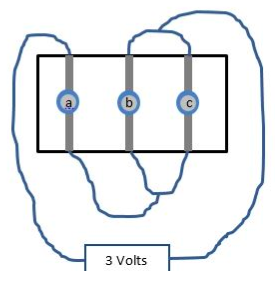# Problem: Consider the circuit with three identical light bulbs shown. What will happen to the brightness of bulbs a and c if bulb b is unscrewed? a. Both bulbs a and c will go out completely b. Both bulbs a and c will remain at the same brightness level that they were before b was unscrewed. c. Bulb a will get brighter and bulb c will get dimmer. d. Bulb a will get dimmer and bulb c will get brighter. e. Bulb a will stay the same but bulb c will get dimmer. f. Bulb a will get brighter and bulb c will stay the same.

###### FREE Expert Solution

In this problem, we are required to combine resistors in series and in parallel.

Equivalent resistance for resistors in parallel:

$\overline{)\frac{\mathbf{1}}{{\mathbf{R}}_{\mathbf{eq}}}{\mathbf{=}}\frac{\mathbf{1}}{{\mathbf{R}}_{\mathbf{1}}}{\mathbf{+}}\frac{\mathbf{1}}{{\mathbf{R}}_{\mathbf{2}}}{\mathbf{+}}{\mathbf{.}}{\mathbf{.}}{\mathbf{.}}{\mathbf{+}}\frac{\mathbf{1}}{{\mathbf{R}}_{\mathbf{n}}}}$

or for 2 resistors:

$\overline{){{\mathbf{R}}}_{{\mathbf{eq}}}{\mathbf{=}}\frac{{\mathbf{R}}_{\mathbf{1}}{\mathbf{R}}_{\mathbf{2}}}{{\mathbf{R}}_{\mathbf{1}}\mathbf{+}{\mathbf{R}}_{\mathbf{2}}}}$

Equivalent resistance for resistors in series:

$\overline{){{\mathbf{R}}}_{{\mathbf{eq}}}{\mathbf{=}}{{\mathbf{R}}}_{{\mathbf{1}}}{\mathbf{+}}{{\mathbf{R}}}_{{\mathbf{2}}}{\mathbf{+}}{\mathbf{.}}{\mathbf{.}}{\mathbf{.}}{\mathbf{+}}{{\mathbf{R}}}_{{\mathbf{n}}}}$

79% (268 ratings)###### Problem Details

Consider the circuit with three identical light bulbs shown. What will happen to the brightness of bulbs a and c if bulb b is unscrewed?a. Both bulbs a and c will go out completely

b. Both bulbs a and c will remain at the same brightness level that they were before b was unscrewed.

c. Bulb a will get brighter and bulb c will get dimmer.

d. Bulb a will get dimmer and bulb c will get brighter.

e. Bulb a will stay the same but bulb c will get dimmer.

f. Bulb a will get brighter and bulb c will stay the same.

Frequently Asked Questions

What scientific concept do you need to know in order to solve this problem?

Our tutors have indicated that to solve this problem you will need to apply the Combining Resistors in Series & Parallel concept. You can view video lessons to learn Combining Resistors in Series & Parallel. Or if you need more Combining Resistors in Series & Parallel practice, you can also practice Combining Resistors in Series & Parallel practice problems.